Queries for Ordered trees: search statistic / browse statistics / browse maps from / browse maps to

# 1. Definition & Example

• An ordered tree is a rooted tree where the children of each node are ordered.

• Equivalently, an ordered tree is recursively defined to be either a leaf (external node) or an ordered list of ordered trees (internal node).

 the 5 Ordered trees of size 4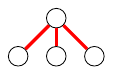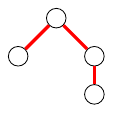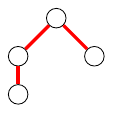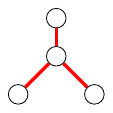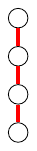[[],[],[]] [[],[[]]] [[[]],[]] [[[],[]]] [[[[]]]]
• There are $\operatorname{Cat}(n) = \frac{1}{n+1}\binom{2n}{n}$ ordered trees with $n+1$ nodes, see OEIS:A000108.